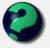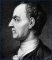Math Is Fun Forum

Discussion about math, puzzles, games and fun.   Useful symbols: ÷ × ½ √ ∞ ≠ ≤ ≥ ≈ ⇒ ± ∈ Δ θ ∴ ∑ ∫  π  -¹ ² ³ °

You are not logged in.

#1 2005-08-12 22:31:40

wcy
Member
Registered: 2005-08-04
Posts: 117

interesting numbers

i found this in a book:

11826²=139854276

30384²=923187456

which are all the digits from 1 to 9

Offline

#2 2005-08-13 10:58:49

NIH
MemberRegistered: 2005-06-14
Posts: 33

Re: interesting numbers

I found this in a book...

1 + 6 + 7 + 17 + 18 + 23 = 2 + 3 + 11 + 13 + 21 + 22;
1^2 + 6^2 + 7^2 + 17^2 + 18^2 + 23^2 = 2^2 + 3^2 + 11^2 + 13^2 + 21^2 + 22^2;
1^3 + 6^3 + 7^3 + 17^3 + 18^3 + 23^3 = 2^3 + 3^3 + 11^3 + 13^3 + 21^3 + 22^3;
1^4 + 6^4 + 7^4 + 17^4 + 18^4 + 23^4 = 2^4 + 3^4 + 11^4 + 13^4 + 21^4 + 22^4;
1^5 + 6^5 + 7^5 + 17^5 + 18^5 + 23^5 = 2^5 + 3^5 + 11^5 + 13^5 + 21^5 + 22^5.

2 + 2 = 5, for large values of 2.

Offline

#3 2005-08-13 11:17:41

mathsyperson
ModeratorRegistered: 2005-06-22
Posts: 4,900

Re: interesting numbers

What an amazing coincidence! It must be coincidence because it stops working when you use any power higher than 5.

Why did the vector cross the road?
It wanted to be normal.

Offline

#4 2005-08-13 11:38:38

NIH
MemberRegistered: 2005-06-14
Posts: 33

Re: interesting numbers

mathsyperson wrote:

What an amazing coincidence! It must be coincidence because it stops working when you use any power higher than 5.

It's a consequence of the following even bigger coincidence!

a^n + (a + 4b + c)^n + (a + b + 2c)^n + (a + 9b + 4c)^n + (a + 6b + 5c)^n + (a + 10b + 6c)^n = (a + b)^n + (a + c)^n + (a + 6b + 2c)^n + (a + 4b + 4c)^n + (a + 10b + 5c)^n + (a + 9b + 6c)^n,

where a, b, c are any positive integers and n can be 1, 2, 3, 4, or 5.

Last edited by NIH (2005-08-13 11:39:19)

2 + 2 = 5, for large values of 2.

Offline

#5 2005-09-05 12:32:55

Zhylliolom
Real MemberRegistered: 2005-09-05
Posts: 412

Re: interesting numbers

Here's an interesting one:

111111111² = 12345678987654321.

Basically, when any number composed entirely of n ones is squared, the result will be 12...n...21. Well, at least when n < 10, once we get to 10 the middle gets screwy.

Last edited by Zhylliolom (2005-09-05 12:33:34)

Offline